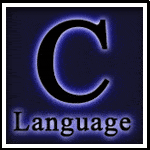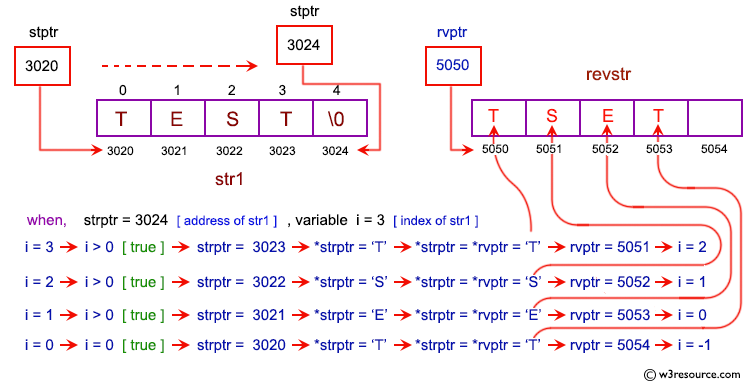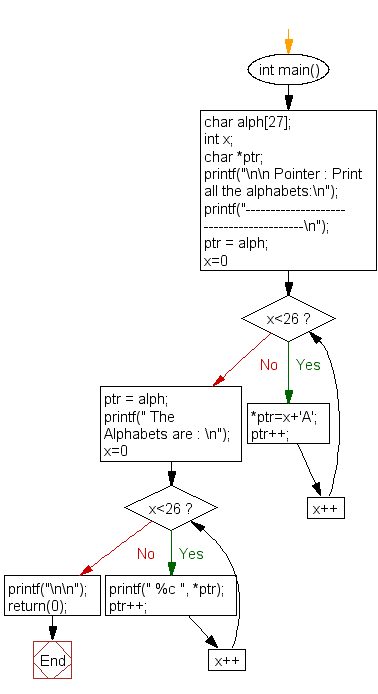﻿ C exercises: Print all the alphabates - w3resource# C Exercises: Print all the alphabets

## C Pointer : Exercise-21 with Solution

Write a program in C to print all the alphabets using pointer.

Pictorial Presentation:Sample Solution:

C Code:

``````#include <stdio.h>

int main()
{
char alph;
int x;
char *ptr;
printf("\n\n Pointer : Print all the alphabets:\n");
printf("----------------------------------------\n");
ptr = alph;

for(x=0;x<26;x++)
{
*ptr=x+'A';
ptr++;
}
ptr = alph;

printf(" The Alphabets are : \n");
for(x=0;x<26;x++)
{
printf(" %c ", *ptr);
ptr++;
}
printf("\n\n");
return(0);
}
```
```

Sample Output:

``` Pointer : Print all the alphabates:
----------------------------------------
The Alphabates are :
A  B  C  D  E  F  G  H  I  J  K  L  M  N  O  P  Q  R  S  T  U  V  W  X  Y  Z
```

Flowchart:C Programming Code Editor:

Have another way to solve this solution? Contribute your code (and comments) through Disqus.

What is the difficulty level of this exercise?

﻿